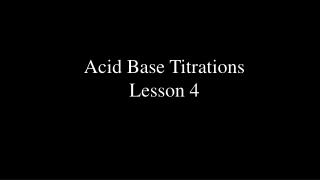# Acid Base Titrations Lesson 4 - PowerPoint PPT PresentationDownload PresentationAcid Base Titrations Lesson 4

Acid Base Titrations Lesson 4Download Presentation## Acid Base Titrations Lesson 4

- - - - - - - - - - - - - - - - - - - - - - - - - - - E N D - - - - - - - - - - - - - - - - - - - - - - - - - - -
##### Presentation Transcript

1. Acid Base Titrations Lesson 4

2. In a titration the molarity of one chemical is determined by reacting it with another one with known molarity (standard).

3. Special Equipment is used for a titration Burette Erlenmeyer Flask Magnetic Stirrer Pipette Pipette Filler

4. Digital Balance Solid acid to make a standard solution Volumetric Flask

5. Titration Calculation 1. 6.50 mL of 0.100 M H2C2O4 is required to neutralize 10.0 mL of KOH solution in a titration. Calculate the base concentration. H2C2O4 + 2KOH  K2C2O4 + 2H2O 0.00650 L 0.0100 L 0.100 M ? M 0.00650 L H2C2O4 x 0.100 mole x 2 mole KOH 1 L 1 mole H2C2O4 [KOH] = 0.0100 L = 0.130 M

6. If you get data in atable, you need to subtractthe finalburette reading from the initialto get the volumeof acid or base added. If you have multiple trials, averagethem but reject any volumes that are way off. Use some common sense. Burette Volume in mL Initial 0.00 mL 8.95 mL 17.41 mL Final 8.95 mL 17.41mL 25.85 mL Volume Added8.95 mL 8.46 mL 8.44 mL reject average 8.46 + 8.44 = 8.45 mL 2

7. Now it's your turn to try one!

8. Titration Calculation 2. 8.95 mL , 8.46 mL and 8.44 mL of 0.200 M H2SO4 was required to neutralize 25.0 mL of KOH solution in a titration. Calculate the base concentration. H2SO4 + 2KOH K2SO4 + 2H2O 0.00845 L 0.0250 L 0.200 M ? M [KOH] = 0.00845 L H2SO4 x 0.200 mole x 2 mole KOH1 L 1 mole H2SO4 0.0250 L = 0.135 M

9. 3. Calculate the mass of H2C2O4.2H2O required to make 100.0 mL of a 0.1000 M standard solution to use in your titration lab tomorrow. 0.100 L x 0.1000 mol x 126.06 g = 1.261 g 1 L 1 mole